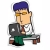## 用SQL做数据分析_1 原

``````select
Top 1000 a.*,
c.service_score
from a,c
where (a.driver_id = c.driver_id)
and (c.service_score between 90 and 100)
and (a.driver_car_lev in ('1','2','3'))
and (a.city_id = 1)``````

``````select
b.*,
COUNT(*) as order1,
SUM(case when (current_lng between 10 and 20) and (current_lan between 100 and 200)  then 1 else 0 end) as order2,
SUM(case when (current_lng not between 10 and 20) or (current_lan not between 100 and 200)  then 1 else 0 end) as order3
from (select
Top 1000 a.*,
c.service_score
from a,c
where (a.driver_id = c.driver_id)
and (c.service_score between 90 and 100)
and (a.driver_car_lev in ('1','2','3'))
and (a.city_id = 1))as d,b
where b.driver_id = d.driver_id
group by b.driver_id,b.order_id,b.current_lng,b.current_lan``````

``````select
driver_id,
COUNT(order1) as tlnum,
SUM(order2) as sqnum,
SUM(order2)*1.0/COUNT(order1) as sqrate,
SUM(order3) as jqnum,
SUM(order3)*1.0/COUNT(order1) as jqrate
from
(select
b.*,
COUNT(*) as order1,
SUM(case when (current_lng between 10 and 20) and (current_lan between 100 and 200)  then 1 else 0 end) as order2,
SUM(case when (current_lng not between 10 and 20) or (current_lan not between 100 and 200)  then 1 else 0 end) as order3
from (select
Top 1000 a.*,
c.service_score
from a,c
where (a.driver_id = c.driver_id)
and (c.service_score between 90 and 100)
and (a.driver_car_lev in ('1','2','3'))
and (a.city_id = 1))as d,b
where b.driver_id = d.driver_id
group by b.driver_id,b.order_id,b.current_lng,b.current_lan) as e
group by driver_id 	``````

``````select
COUNT(*) as tldriver,
SUM(case when (sqrate > 0.5) then 1 else 0 end) as sqdriver,
SUM(case when (sqrate > 0.5) then 1 else 0 end)*1.0/COUNT(*) as sqdeiverrate,
SUM(case when (jqrate > 0.5) then 1 else 0 end) as jqdriver,
SUM(case when (jqrate > 0.5) then 1 else 0 end)*1.0/COUNT(*) as jqdeiverrate
from
(select
driver_id,
COUNT(order1) as tlnum,
SUM(order2) as sqnum,
SUM(order2)*1.0/COUNT(order1) as sqrate,
SUM(order3) as jqnum,
SUM(order3)*1.0/COUNT(order1) as jqrate
from
(select
b.*,
COUNT(*) as order1,
SUM(case when (current_lng between 10 and 20) and (current_lan between 100 and 200)  then 1 else 0 end) as order2,
SUM(case when (current_lng not between 10 and 20) or (current_lan not between 100 and 200)  then 1 else 0 end) as order3
from (select
Top 1000 a.*,
c.service_score
from a,c
where (a.driver_id = c.driver_id)
and (c.service_score between 90 and 100)
and (a.driver_car_lev in ('1','2','3'))
and (a.city_id = 1))as d,b
where b.driver_id = d.driver_id
group by b.driver_id,b.order_id,b.current_lng,b.current_lan) as e
group by driver_id) as f 	``````

①使用对象资源管理器创建表a,b,c

②使用C#批量插入数据

``````using System;
using System.Collections.Generic;
using System.Data;
using System.Data.SqlClient;
using System.Linq;
using System.Text;

namespace DD
{
class Program
{

static void Main(string[] args)
{
String CONNECTION_STRING = "server = .;database=DD;Integrated Security = true;";

SqlConnection conn = new SqlConnection(CONNECTION_STRING);
conn.Open();

SqlCommand cmd = new SqlCommand();
cmd.Connection = conn;
cmd.CommandType = CommandType.Text;

for (int i = 1; i <=1000; i++)
{
string sql =
"insert into a(driver_id, city_id, driver_car_lev) values (" + Convert.ToString(i) + "," + Convert.ToString(1) + "," + Convert.ToString(1) + ")";
cmd.CommandText = sql;
cmd.ExecuteNonQuery();
}

for(int i =1; i<=600; i++){
for (int j = 1; j <= 3; j++)
{
string sql =
"insert into b(driver_id, order_id, current_lng, current_lan) values (" + Convert.ToString(i) + "," + Convert.ToString(j) + "," + Convert.ToString(new Random().Next(10) + 10) + "," + Convert.ToString(new Random().Next(100) + 100) + ")";
cmd.CommandText = sql;
cmd.ExecuteNonQuery();
}
for (int j = 4; j <= 4; j++)
{
string sql =
"insert into b(driver_id, order_id, current_lng, current_lan) values (" + Convert.ToString(i) + "," + Convert.ToString(j) + "," + Convert.ToString(new Random().Next(10) + 30) + "," + Convert.ToString(new Random().Next(100) + 300) + ")";
cmd.CommandText = sql;
cmd.ExecuteNonQuery();
}
}
for (int i = 601; i <= 1000; i++)
{
for (int j = 1; j <= 3; j++)
{
string sql =
"insert into b(driver_id, order_id, current_lng, current_lan) values (" + Convert.ToString(i) + "," + Convert.ToString(j) + "," + Convert.ToString(new Random().Next(10) + 30) + "," + Convert.ToString(new Random().Next(100) + 300) + ")";
cmd.CommandText = sql;
cmd.ExecuteNonQuery();
}
for (int j = 4; j <= 4; j++)
{
string sql =
"insert into b(driver_id, order_id, current_lng, current_lan) values (" + Convert.ToString(i) + "," + Convert.ToString(j) + "," + Convert.ToString(new Random().Next(10) + 10) + "," + Convert.ToString(new Random().Next(100) + 100) + ")";
cmd.CommandText = sql;
cmd.ExecuteNonQuery();
}
}

for (int i = 1; i <= 1000; i++)
{
string sql =
"insert into c(driver_id, service_score) values (" + Convert.ToString(i) + "," + Convert.ToString(new Random().Next(10) + 90) + ")";
cmd.CommandText = sql;
cmd.ExecuteNonQuery();
}

//System.Console.Write("column1\tcolumn2\tcolumn3\n");
//{
//}
conn.Close();
System.Console.Write("Insert complete!");

}
}
}
``````

③测试SQL脚本

``````select
COUNT(*) as tldriver,
SUM(case when (sqrate > 0.5) then 1 else 0 end) as sqdriver,
SUM(case when (sqrate > 0.5) then 1 else 0 end)*1.0/COUNT(*) as sqdeiverrate,
SUM(case when (jqrate > 0.5) then 1 else 0 end) as jqdriver,
SUM(case when (jqrate > 0.5) then 1 else 0 end)*1.0/COUNT(*) as jqdeiverrate
from
(select
driver_id,
COUNT(order1) as tlnum,
SUM(order2) as sqnum,
SUM(order2)*1.0/COUNT(order1) as sqrate,
SUM(order3) as jqnum,
SUM(order3)*1.0/COUNT(order1) as jqrate
from
(select
b.*,
COUNT(*) as order1,
SUM(case when (current_lng between 10 and 20) and (current_lan between 100 and 200)  then 1 else 0 end) as order2,
SUM(case when (current_lng not between 10 and 20) or (current_lan not between 100 and 200)  then 1 else 0 end) as order3
from (select
Top 1000 a.*,
c.service_score
from a,c
where (a.driver_id = c.driver_id)
and (c.service_score between 90 and 100)
and (a.driver_car_lev in ('1','2','3'))
and (a.city_id = 1))as d,b
where b.driver_id = d.driver_id
group by b.driver_id,b.order_id,b.current_lng,b.current_lan) as e
group by driver_id) as f 	``````

Over！！！### 评论(2)#### 引用来自“lijiachen56”的评论

SUM(order2)*1.0/COUNT(order1) as sqrate

l

SUM(order2)*1.0/COUNT(order1) as sqrate

wx5e96c1530227f
05/20
0
0

2018/11/05
0
0

2018/07/13
1
0

2018/07/13
0
0

2019/10/21
0
0

flydean
22分钟前
12
1

// ==UserScript==// @name 直接显示StackOverflow的答题日期， 增加评论区回复的时间显示 ，修改时间显示到小时分。// @namespace http://tampermonkey.net/// @version ...

FalconChen

36
0

STONE-CITY

12
0
iOS MVVM 与RAC结合使用

MVVM配合 RAC 更能发挥的淋漓尽致。 我们把 MVVM 第一篇的例子 KVO 的事件 替换成 配合RAC 框架使用， OC的话直接导入 ： pod 'ReactiveObjC' Swift 直接用 RXSwift就可以。 把 ViewModel里加...

T型人才追梦者

22
1
OSChina 周一乱弹 —— 影响心情的三座大山

Osc乱弹歌单（2020）请戳（这里） 【今日歌曲】 @薛定谔的兄弟 ：分享洛神有语创建的歌单「我喜欢的音乐」: 《浮生(inst.)》- 忘乡 / 墨凡悦 手机党少年们想听歌，请使劲儿戳（这里） @凝小紫...

108
2